#### Jackknife Method

The jackknife method of variance estimation deletes one PSU at a time from the full sample to create replicates. The total number of replicates R is the same as the total number of PSUs. In each replicate, the sample weights of the remaining PSUs are modified by the jackknife coefficient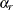. The modified weights are called replicate weights.

The jackknife coefficient and replicate weights are described as follows.

##### Without Stratification

If there is no stratification in the sample design (no STRATA statement), the jackknife coefficientsare the same for all replicates: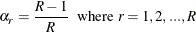Denote the original weight in the full sample for the jth member of the ith PSU as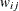. If the ith PSU is included in the rth replicate (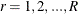), then the corresponding replicate weight for the jth member of the ith PSU is defined as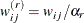##### With Stratification

If the sample design involves stratification, each stratum must have at least two PSUs to use the jackknife method.

Let stratum
be the stratum from which a PSU is deleted for the rth replicate. Stratumis called the donor stratum. Let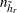be the total number of PSUs in the donor stratum. The jackknife coefficients are defined as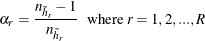Denote the original weight in the full sample for the jth member of the ith PSU as. If the ith PSU is included in the rth replicate (), then the corresponding replicate weight for the jth member of the ith PSU is defined as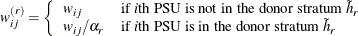You can use the VARMETHOD=JACKKNIFE(OUTJKCOEFS=) method-option to save the jackknife coefficients into a SAS data set and use the VARMETHOD=JACKKNIFE(OUTWEIGHTS=) method-option to save the replicate weights into a SAS data set.

If you provide your own replicate weights with a REPWEIGHTS statement, then you can also provide corresponding jackknife coefficients with the JKCOEFS= option.

Let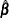be the estimated regression coefficients from the full sample for. Let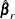be the estimated regression coefficient obtained from the rth replicate by using replicate weights. PROC SURVEYREG estimates the covariance matrix ofby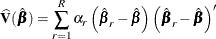with RH degrees of freedom, where R is the number of replicates and H is the number of strata, or R–1 when there is no stratification.